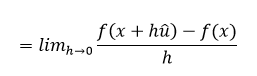# Directional Derivative

The directional derivative is the rate at which any function changes at any particular point in a fixed direction. It is a vector form of any derivative. It characterizes the instantaneous rate of modification of the function. It generalizes the view of a partial derivative. It can be defined as:

uf ≡ ▽f.(u/|u|)In this article, we shall understand the concept of directional derivative in detail. We shall learn its definition, formula, gradient and properties. We will go ahead and learn about the normal derivative concept also. We will also discuss a few solved examples of calculating the directional derivative.

## Directional Derivative Definition

For a scalar function f(x)=f(x1,x2,…,xn), the directional derivative is defined as a function in the following form;

uf = limh→0[f(x+hv)-f(x)]/h

Where v be a vector along which the directional derivative of f(x) is defined. Sometimes, v is restricted to a unit vector, but otherwise, also the definition holds.

Vector v is given by;

v = (v1,v2,v3,…,vn)

### Properties of Directional Derivative

The basic properties related to the directional derivative are discussed below. Suppose any two functions f and g are defined in a neighbourhood of a point ‘a’ and are differentiable at ‘a’.

1. Rule for Constant Factor
2. Let k be a constant, then;

v(kf)=k▽vf

3. Rule for Sum
4. The sum is distributive.

v(f+g)=▽vf+▽vg

5. Rule for Product
6. This is also known as Leibniz’s rule.

v(fg) = g▽vf + f▽vg

7. Chain Rule
8. It applies when f is differentiable at ‘a’ and g is differentiable at f(a). In such a case,

v(f o g)(a) = f′(g(a)) ▽vg(a)

### Formula

The directional derivative is defined as n.▽f. Here, n is considered as a unit vector. The directional derivative is defined as the rate of change along the path of the unit vector which is u = (a,b). The directional derivative is denoted by Du f(x,y) which can be written as follows:

 Duf(x,y) = limh→0[f(x+ah,y+bh)-f(x,y)]/h

### Example Problem

Q.1: Find the directional derivative of the function f(x,y) = xyz in the direction 3i – 4k. It has the points as (1,-1,1).

Solution: Given function is f(x,y) = xyz

Vector field is 3i – 4k. It has the magnitude of √[(32)+(−42) = √25 = √5

The unit vector n in the direction 3i – 4k is thus n = 1/5(3i−4k)

Now, we have to find the gradient ▽f for finding the directional derivative.

Thus, ▽f = yzi + xzj + xyk

Now, the directional derivative is;

n.▽f = 1/5 (3i−4k).(yzi+xzj+xyk)

= 1/5 [3 × yz + 0 − 4 × xy]

The directional derivative at the point (1,-1,1) is;

n.▽f = 1/5[3×(−1)×(1)−4×1×(−1)]

n.▽f = ⅕

Since we know that the gradient is defined for the function f(x,y) is as;

▽f = ▽f(x,y) = ∂f/∂xi + ∂f/∂yj

This can be calculated by assigning the vector operator r to the f(x,y) which is a scalar function. That vector field is known as a gradient vector field.

If we have a function f(x,y,z) and u(u1, u2, u3) is the unit vector, then;

Duf = ▽f.u = ∂f/∂x u1 + ∂f/∂y u2 + ∂f/∂z u3

It is clear that, if we take a dot product of the gradient and the given unit vector, then we get the directional derivative of the function.

Example: Find the gradient of the function f(x,y) = x + y.

Solution: Given function is f(x,y) = x + y

▽f = ▽f(x,y) = (∂f/∂x)i + (∂f/∂y)j

▽f = [∂(x+y)/∂x]i + [∂(x+y)/∂y]j

▽f = (1 + 0)i + (0 + 1)j

▽f = i + j

Therefore, the gradient of the function f(x,y) = x + y is i + j.